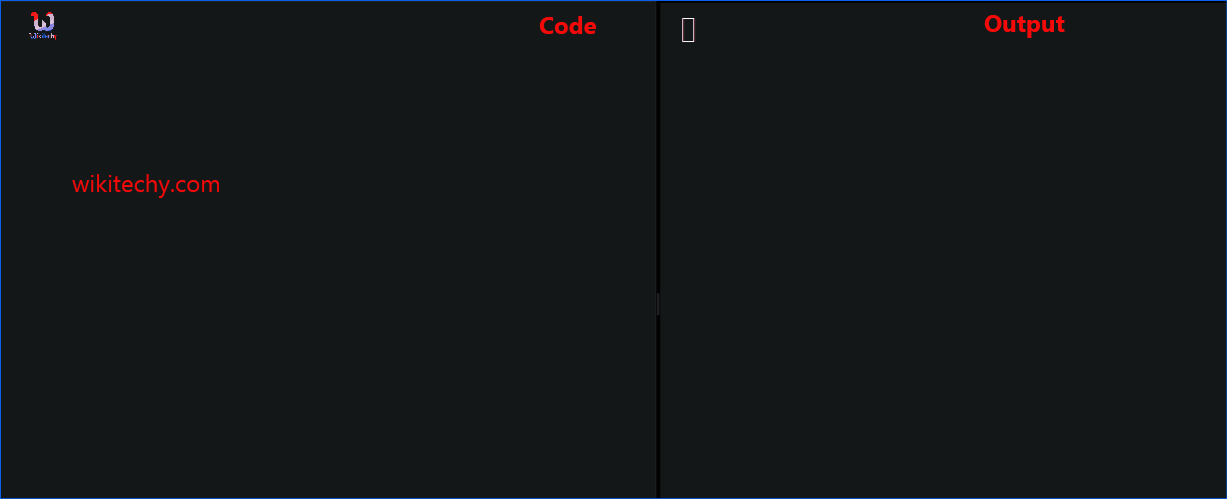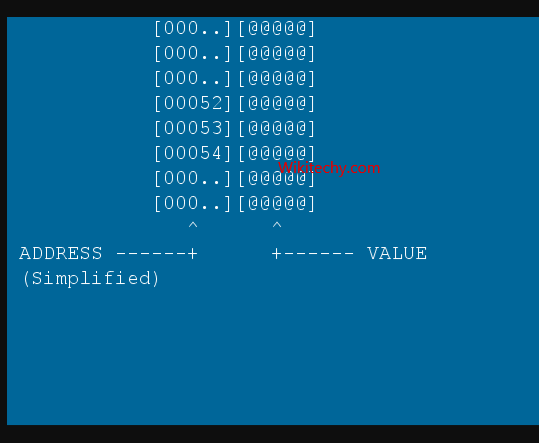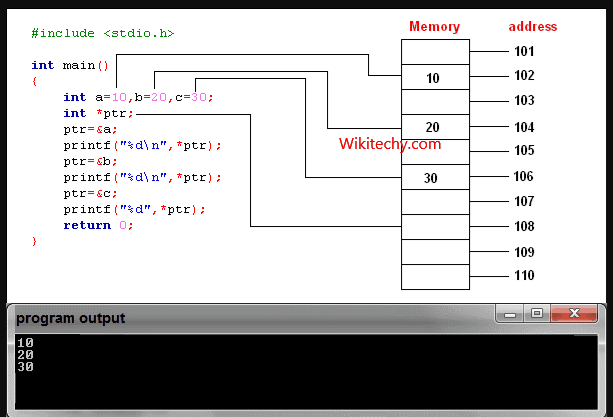# Pointer to Structure | C++ Pointers to Structure - Learn C++ - C++ Tutorial - C++ programmingLearn c++ - c++ tutorial - c++-pointers-to-structure - c++ examples - c++ programs

## What is Pointers in C++

• A pointer is a variable that holds a memory address. This address is the location of another object (typically, a variable) in memory. That is, if one variable contains the address of another variable, the first variable is said to point to the second.
•learn c++ tutorials - pointers in c++ Example

• A pointer declaration consists of a base type, an *, and the variable name.
• The general form of declaring a pointer variable is:
type *name;
• The 'type' is the base type of the pointer and may be any valid type.
• The 'name' is the name of pointer variable.
• The base type of the pointer defines what type of variables the pointer can point to.
• A pointer variable can be created not only for native types like (int, float, double etc.) but they can also be created for user defined types like structure.
• If you do not know what pointers are, visit C++ pointers.
•learn c++ tutorials - pointers in c++ Example

• Here is how you can create pointer for structures:
• This program creates a pointer ptr of type structure temp.

## Output

• In this program, a pointer variable ptr and normal variable d of type structure Distance is defined.
• The address of variable d is stored to pointer variable, that is, ptr is pointing to variabled. Then, the member function of variable d is accessed using pointer.
• Note: Since pointer ptr is pointing to variable d in this program, (*ptr).inch and d.inch is exact same cell. Similarly, (*ptr).feet and d.feet is exact same cell.
• The syntax to access member function using pointer is ugly and there is alternative notation-> which is more common.